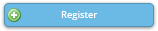Legend:
CC = Baltimore Convention Center,    H = Hilton Baltimore
* = applied session       ! = JSM meeting theme

#### Activity DetailsTweet CE_32T Wed, 8/2/2017, 10:00 AM - 11:45 AM H-Holiday Ballroom 1 Bayesian Analysis Using Stata (ADDED FEE) — Professional Development Computer Technology Workshop ASA , Stata This workshop covers the use of Stata to perform Bayesian analysis. Bayesian analysis is a statistical paradigm that answers research questions about unknown parameters using probability statements. For example, what is the probability that people in a particular state vote Republican or vote Democrat? What is the probability that a person accused of a crime is guilty? What is the probability that the odds ratio is between 0.3 and 0.5? And many more. Such probabilistic statements are natural to Bayesian analysis because of the underlying assumption that all parameters are random quantities. In Bayesian analysis, a parameter is summarized by an entire distribution of values instead of one fixed value as in classical frequentist analysis. Estimating this distribution, a posterior distribution of a parameter of interest, is at the heart of Bayesian analysis. This workshop will demonstrate the use of Bayesian analysis in various applications and will introduce Stata's suite of commands for conducting Bayesian analysis. No prior knowledge of Stata is required, but basic familiarity with Bayesian analysis will prove useful. Instructor(s): Yulia Marchenko, StataCorp LP

Copyright © American Statistical Association